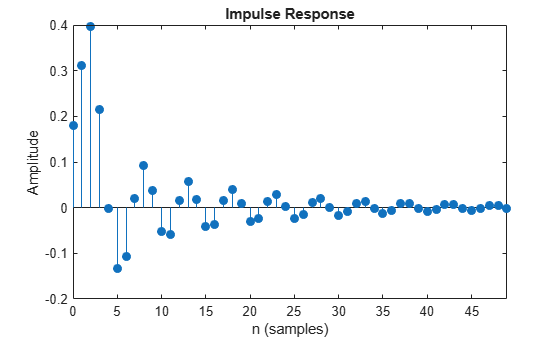impz

Impulse response of digital filter

Description

example

[h,t] = impz(b,a) returns the impulse response of the digital filter with numerator coefficients b and denominator coefficients a. The function chooses the number of samples and returns the response coefficients in h and the sample times in t.

[h,t] = impz(sos) returns the impulse response of the filter specified by the second-order sections matrix sos.

example

[h,t] = impz(d) returns the impulse response of the digital filter d. Use designfilt to generate d based on frequency-response specifications.

example

[h,t] = impz(___,n) specifies what impulse-response samples to compute. You can specify the filter using any of the previous syntaxes.

example

[h,t] = impz(___,n,fs) returns a vector t with consecutive samples spaced 1/fs units apart.

example

impz(___) with no output arguments plots the impulse response of the filter.

Examples

collapse all

Design a fourth-order lowpass elliptic filter with normalized passband frequency 0.4 rad/sample. Specify a passband ripple of 0.5 dB and a stopband attenuation of 20 dB. Plot the first 50 samples of the impulse response.

[b,a] = ellip(4,0.5,20,0.4);
impz(b,a,50)Design the same filter using designfilt. Plot the first 50 samples of its impulse response.

d = designfilt('lowpassiir','DesignMethod','ellip','FilterOrder',4, ...
'PassbandFrequency',0.4, ...
'PassbandRipple',0.5,'StopbandAttenuation',20);
impz(d,50)Design an FIR highpass filter of order 18 using a Kaiser window with $\beta =4$. Specify a sample rate of 100 Hz and a cutoff frequency of 30 Hz. Display the impulse response of the filter.

b = fir1(18,30/(100/2),'high',kaiser(19,4));
impz(b,1,[],100)Design the same filter using designfilt and plot its impulse response.

d = designfilt('highpassfir','FilterOrder',18,'SampleRate',100, ...
'CutoffFrequency',30,'Window',{'kaiser',4});
impz(d,[],100)Input Arguments

collapse all

Transfer function coefficients, specified as vectors. Express the transfer function in terms of b and a as

$H\left({e}^{j\omega }\right)=\frac{B\left({e}^{j\omega }\right)}{A\left({e}^{j\omega }\right)}=\frac{\text{b(1)}+\text{b(2)}\text{\hspace{0.17em}}{e}^{-j\omega }+\text{b(3)}\text{\hspace{0.17em}}{e}^{-j2\omega }+\cdots +\text{b(M)}\text{\hspace{0.17em}}{e}^{-j\left(M-1\right)\omega }}{\text{a(1)}+\text{a(2)}\text{\hspace{0.17em}}{e}^{-j\omega }+\text{a(3)}\text{\hspace{0.17em}}{e}^{-j2\omega }+\cdots +\text{a(N)}\text{\hspace{0.17em}}{e}^{-j\left(N-1\right)\omega }}.$

Example: b = [1 3 3 1]/6 and a = [3 0 1 0]/3 specify a third-order Butterworth filter with normalized 3 dB frequency 0.5π rad/sample.

Data Types: double | single
Complex Number Support: Yes

Second-order section coefficients, specified as a matrix. sos is a K-by-6 matrix, where the number of sections, K, must be greater than or equal to 2. If the number of sections is less than 2, the function treats the input as a numerator vector. Each row of sos corresponds to the coefficients of a second-order (biquad) filter. The ith row of sos corresponds to [bi(1) bi(2) bi(3) ai(1) ai(2) ai(3)].

Example: s = [2 4 2 6 0 2;3 3 0 6 0 0] specifies a third-order Butterworth filter with normalized 3 dB frequency 0.5π rad/sample.

Data Types: double | single
Complex Number Support: Yes

Digital filter, specified as a digitalFilter object. Use designfilt to generate a digital filter based on frequency-response specifications.

Example: d = designfilt('lowpassiir','FilterOrder',3,'HalfPowerFrequency',0.5) specifies a third-order Butterworth filter with normalized 3 dB frequency 0.5π rad/sample.

Sample numbers, specified as a positive integer, a vector of nonnegative integers, or an empty vector.

• If n is a positive integer, impz computes the first n samples of the impulse response and returns t as (0:n-1)'.

• If n is a vector of nonnegative integers, impz computes the impulse response at the locations specified in the vector.

• If n is an empty vector, impz computes the number of samples automatically. See Algorithms for more information.

Example: impz([2 4 2 6 0 2;3 3 0 6 0 0],5) computes the first five samples of the impulse response of a Butterworth filter.

Example: impz([2 4 2 6 0 2;3 3 0 6 0 0],[0 3 2 1 4 5]) computes the first six samples of the impulse response of a Butterworth filter.

Example: impz([2 4 2 6 0 2;3 3 0 6 0 0],[],5e3) computes the impulse response of a Butterworth filter designed to filter signals sampled at 5 kHz.

Sample rate, specified as a positive scalar. When the unit of time is seconds, fs is expressed in hertz.

Data Types: double

Output Arguments

collapse all

Impulse response coefficients, returned as a column vector.

Sample times, returned as a column vector.

Algorithms

impz filters a length-n impulse sequence using

filter(b,a,[1 zeros(1,n-1)])

and plots the result using stem.

Note

If the input to impz is single precision, the function computes the impulse response using single-precision arithmetic and returns single-precision output.

When impz calculates n automatically, the algorithm depends on the properties of the filter:

• FIR filters — n is the length of b.

• IIR filters — impz first finds the poles of the transfer function using roots.

• If the filter is unstable, n is chosen to be the point at which the term from the largest pole reaches 106 times its original value.

• If the filter is stable, n is chosen as the point at which the term from the largest-amplitude pole is 5 × 10–5 times its original amplitude.

• If the filter is oscillatory with poles on the unit circle only, impz computes five periods of the slowest oscillation.

• If the filter has both oscillatory and damped terms, n is the greater of five periods of the slowest oscillation, or the point at which the term due to the largest pole is 5 × 10–5 times its original amplitude.

impz also allows for delays in the numerator polynomial. The number of delays is incorporated into the computation of the number of samples.